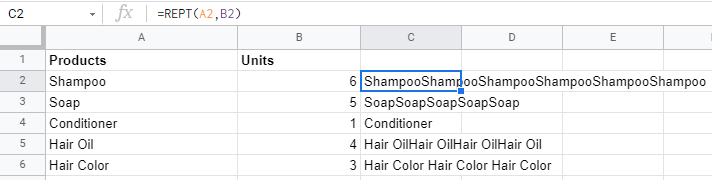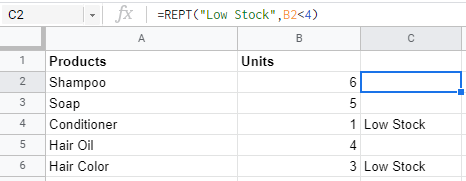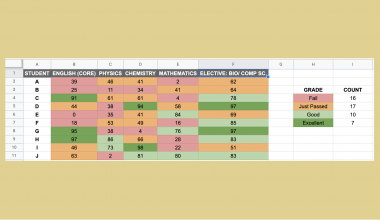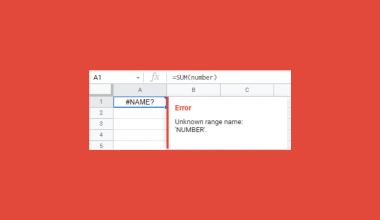# How to Use REPT Function in Google Sheets

The REPT function in Google Sheets is useful if you want to repeat a text several times.

The function returns a set number of texts listed within the google sheets.

#### Let’s take an example:

There are many ways to use the `REPT` function, but here are the two main ways to show an easy demonstration.

#### Example 1:

As you can see, A2 is the given text that we want to repeat, B2 is the number of times we want to repeat the text.

By using the REPT function, C2 would return the word “Shampoo” six times.#### Example 2:

You can also use the function by directly inputting the `text` you want to show as a result.

By inputting “|” directly into the formula, the function will return the amount of “|” implicated in B2.#### Example 3:

The `REPT` function can also be used with many other functions such as LEN, SPLIT, ArrayFormula, JOIN, and many more!

Here is an example to show you how to incorporate `SPLIT` function into `REPT` function:By adding the `SPLIT` function, it makes the `REPT` function the `text` in the formula, followed by the `delimeter`, a character we use to split the text into different columns.

This formula will return each “|” in different columns.

#### Example 4:

You can also use `REPT` function just like an `IF` function in some situations as a logical formula.To have a more visual spreadsheet to analyze which items are in need of restocking, we can use the `REPT` function to only show the items that are low in stock.

Instead of only putting the numbers to repeat, we put in a logical argument to only show “Low Stock” if the item is left with less than 4 units.

## The Anatomy of the REPT Function

The way we write the REPT function is:

`=REPT(text_to_repeat, number_of_repititions)`

• The equal sign `=`  is how we start any function in Google Sheets.
• `REPT()` is our function. We need to add two attributes, namely the `text_to_repeat` and `number_of_repitition`, to make it work correctly.
• The `text_to_repeat` is the text/ character to repeat.
• The `number_of_repitition` is the number of times `text_to_repeat` should appear.

#### Let’s take note that:

The `REPT` function does not insert spaces between repetitions of the `text_to_repeat`. If the spacing is desired, a space must be inserted to the end value of the `text_to_repeat`.

## A Real Life Example of Using REPT Function

The `REPT` function is often used as a visualization for ease of understanding a set of data, such as this:By using the `REPT` function, you can easily tell which pastries are the best-selling items!

You can create many different styles of in-cell charts to beautify your Google Sheet! Sweet!👌

## How to Use REPT Function in Google Sheets

#### Example 1:

1. Simply click on the cell that you want to write down your function at. In this example, it will be C2.1. Begin your function with an equal sign `=`, followed by the name of the function, `REPT`, then an open parenthesis `(`.1. We will then add the character that we want to add. In our case, it’s the 🥐 emoji. To access the Emoji Keyboard Panel, you can simply press the “Window key” + “.” simultaneously. This would then appear:1. Next, enclosed by a quote-unquote symbol `""`, type in the character we want to repeat.1. Then, we will add another comma `,` to separate the `text_to_repeat` from the `number_of_repitition` which will be B2. We end the formula by closing it with a parenthesis `)`.Our final formula would look like this:

`=REPT(“🥐”,B2)`

1. After the following steps, your input should look like this.You may make a copy of the spreadsheet using the link attached below and try it for yourself:

There you go! A fun way to show your data! 💪

Do subscribe to us for more upcoming interesting Google Sheets and Excel function tutorials!

##### You May Also Like## How to Count Cells Based on the Cell Color in Google Sheets

While there are no built-in functions in Google sheets to count cells based on the cell color, you…## How to Use DVAR Function in Google Sheets

The DVAR function in Google Sheets is useful if you need to estimate the variance of a population,…## How to Use ARRAYFORMULA Function in Google Sheets

The ARRAYFORMULA function in Google Sheets is useful to apply a formula to an entire column in Google…Removing hyperlinks is useful when you want to remove texts automatically recognized by Google Sheets as hyperlinks. First,…